# Performing Early Warning Signal Assessments

#### 2023-04-25

This tutorial introduces how to perform both univariate and multivariate early warning signal (EWS) assessments using `EWSmethods`. It will give examples of rolling and expanding window approaches for univariate data, introduce trait-based composite EWSs and then conclude with an example of multivariate EWSs.

Greater detail on each function can be found at the Reference page.

# Getting started

``````set.seed(123) #to ensure reproducible data

library(EWSmethods)``````

## 1. The data

`EWSmethods` comes bundled with two data objects which allow you to practice using the `uniEWS()` and `multiEWS()` functions in both transitioning and non-transitioning data before applying it to your own use case.

`"simTransComms"` contains three replicate datasets of a simulated five species community that has been driven to transition by the introduction of an invasive species (following Dakos 2018). This will be our multivariate dataset when using `multiEWS()` although we may also use each time series in isolation in `uniEWS()`.

`"CODrecovery"` contains three replicate datasets of a simulated cod ( Gadus morhua ) population that transitions from an overfished to a recovered state following the relaxation of fishing pressure. This data was first published by Clements et al. 2019. While univariate, `"CODrecovery"` provides extra information on the body size of cod individuals which will improve composite EWSs estimated by `uniEWS()`.

``````#Load the two datasets in to the session
data("simTransComms")

data("CODrecovery")``````

We can visualise a community from each of these datasets using the code below:

``matplot(simTransComms\$community1[,3:7], type = "l", xlab = "Time", ylab = "Density",  main = "Transitioning five species community")``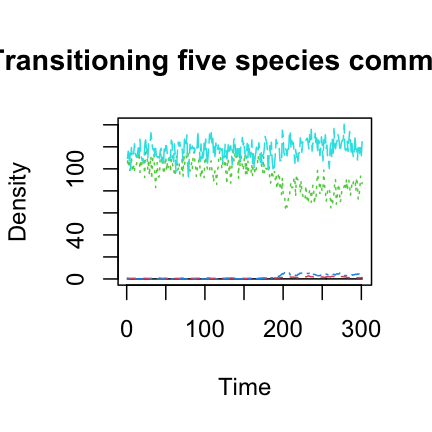``````
plot(x = CODrecovery\$scenario2\$time, y = CODrecovery\$scenario2\$biomass, type = "l", xlab = "Year", ylab = "Abundance", main = "Recovering cod population")``````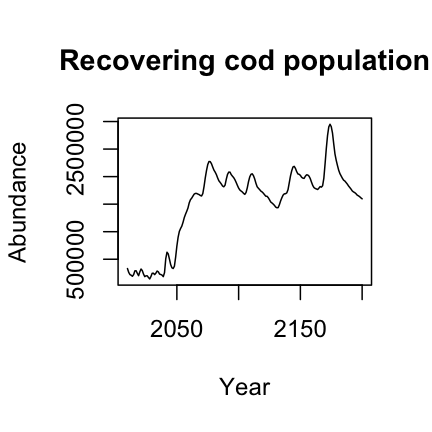These plots show that a transition takes place at `time ~= 180` in `"simTransComms\$community1"` and `year ~= 2050` in `"CODrecovery\$scenario2"`. `EWSmethods` helpfully provides this information in each dataset under the `inflection_pt` column.

simTransComms CODrecovery
191.5 2055

However, EWS assessments are only meaningful if performed on data prior to a transition. As `EWsmethods` provides the time point of transition for both datasets, we can truncate our time series to pre-transition data only.

``````pre_simTransComms <- subset(simTransComms\$community1,time < inflection_pt)

pre_CODrecovery <- subset(CODrecovery\$scenario2,time < inflection_pt)``````

In reality, EWSs will be assessed in real-time with the presence of past/present tipping points often unknown. If past transitions are known to have occurred, it may be prudent to follow the suggestions of O’Brien & Clements (2021) who show that the occurrence of a historic transition can mask an oncoming event and that only using data post the historic transition improves EWS reliability.

Now the data has been loaded and truncated, it can now be passed to `uniEWS()` and `multiEWS()` to perform EWS assessments.

## 2. Univariate EWSs

`EWSmethods` provides two computational approaches to calculate univariate EWSs via the `uniEWS()` function - rolling vs expanding windows. The difference between the two is evident in the figure below but simply, rolling windows estimate EWSs in subsets of the overall time series before ‘rolling’ on one data point and reassessing, Conversely, expanding windows add data points sequentially in to an ‘expanding’ assessment and then standardises against the running mean and standard deviation of the previous window.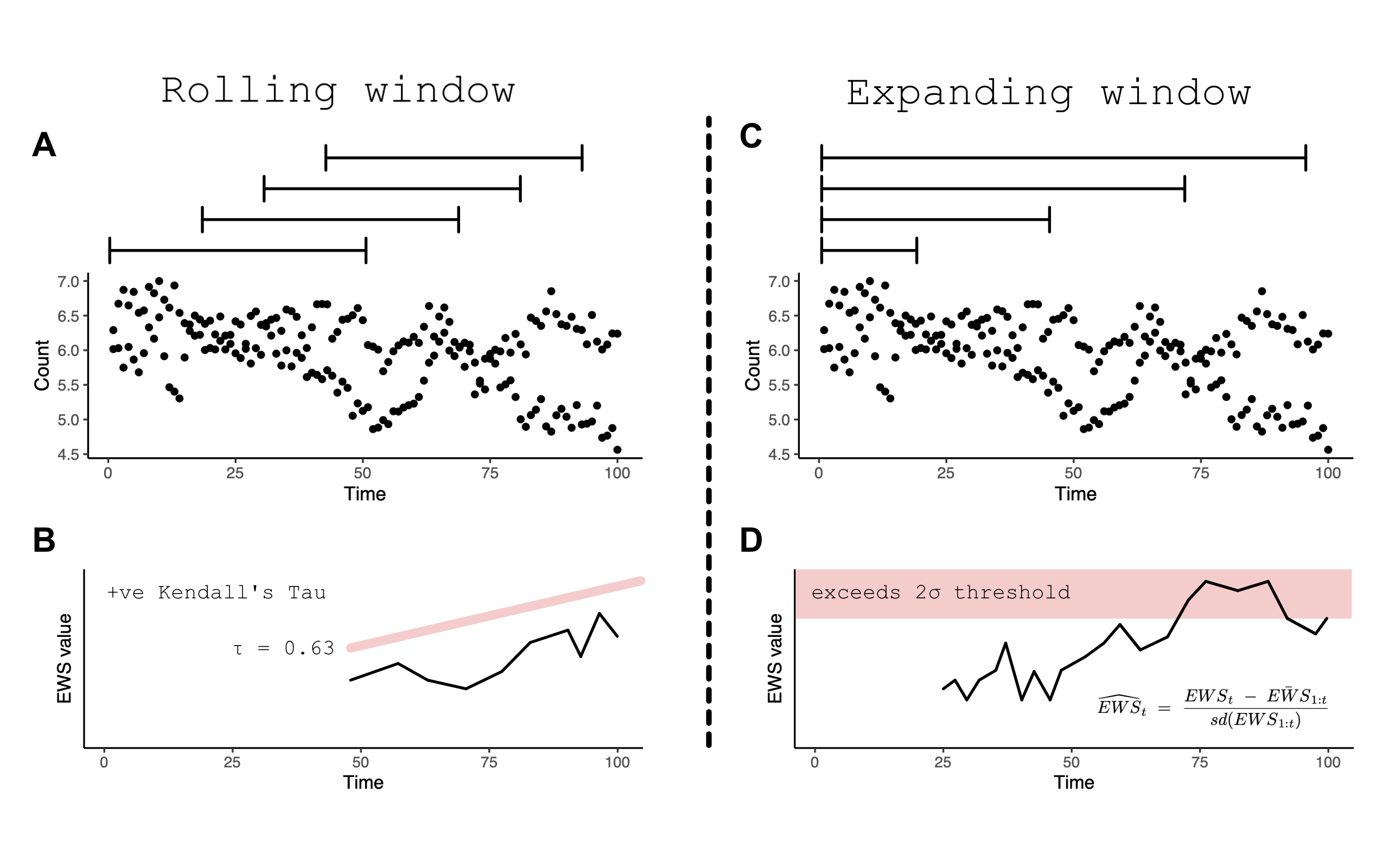Rolling (A) vs expanding (C) window concept diagram. Panels B and D introduce the circumstance when a ‘warning’ is signalled in both approaches

Both computational approaches are able to calculate the same EWS indicators. A brief outline of each can be found in the following table as well as their reference code in `uniEWS()` for the `metrics =` argument.

EWS indicator Description `uniEWS()` metric code
Standard deviation Increasing variance/standard deviation is observed approaching a transition `"SD"`
Coefficient of variation Equivalent to SD as is simply SD at time t divided by the mean SD of the time series `"cv"`
Autocorrelation at lag1 Autocorrelation (similarity between successive observations) increases approaching a transition. The value of this indicator can be estimated as either the autocorrelation coefficient estimated from a first order autoregressive model or the estimated autocorrelation function at lag1 `"ar1"` - autoregressive model, `"acf"`- autocorrelation function
Skewness At a transition, the distribution of values in the time series can become asymmetric `"skew"`
Kurtosis Kurtosis represents the system reaching more extreme values in the presence of a transition. Due to the increased presence of rare values in the time series, the tails of the observation distribution widen `"kurt"`
Return rate The inverse of the first-order term of a fitted autoregressive AR(1) model. Return rate is the primary quantity impacted by CSD – return rate decreases as a tipping point is approached `"rr"`
Density ratio Spectral reddening (high variance at low frequencies) occurs near transition. The density ratio quantifies the degree of reddening as the ratio of the spectral density at low frequency to the spectral density at high frequency `"dr"`

### Rolling windows

The rolling window approach is the most commonly used form of EWS computation due to the work of Dakos et al 2012 and the `earlywarnings` package. `uniEWS()` accepts a `method =` and a `winsize =` argument which calls the expanding window method and creates a rolling window `winsize`% of the total time series length.

Lets use an example where we are interested in the autocorrelation, variance and skewness of one of the five species in `pre_simTransComms`. First we supply a dataframe/matrix of n x 2 dimensions (first column is an equally time sequence and the second is the abundance/biomass time series) and the EWS indicator metrics. The remaining arguments specify the form of computation, window size and plotting characteristics.

``````rolling_ews_eg <- uniEWS(data = pre_simTransComms[,c(2,5)],
metrics = c("ar1","SD","skew"),
method = "rolling",
winsize = 50)``````
``plot(rolling_ews_eg,  y_lab = "Density")``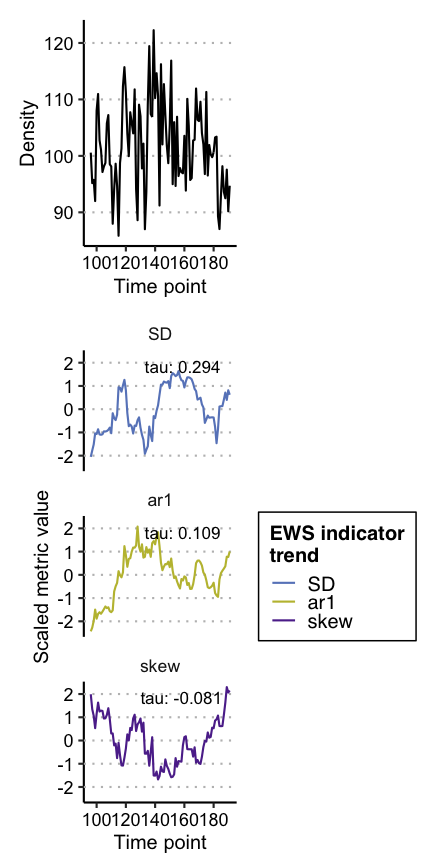Note how all EWS indicators begin to trend upwards at `time ~= 170` which results in the positive Kendall Tau correlation coefficient indicative of an oncoming transition/tipping point.

### Expanding windows

Let’s explore the alternative expanding window approach. All we need to change in `uniEWS()` is the `method =` argument, and replace `winsize =` with `burn_in =`. Instead of specifying the size of the rolling window, `burn_in =` dictates the number of datapoints `uniEWS()` is to use to ‘train’ the algorithm. This mitigates the high number of false-positive signals resulting from the short time series length and high variability when few data points are supplied at the beginning of assessment (O’Brien & Clements, 2021).

``````expanding_ews_eg <- uniEWS(data = pre_simTransComms[,c(2,5)],
metrics = c("ar1","SD","skew"),
method = "expanding",
burn_in = 50,
threshold = 2)``````
``plot(expanding_ews_eg, y_lab = "Density")``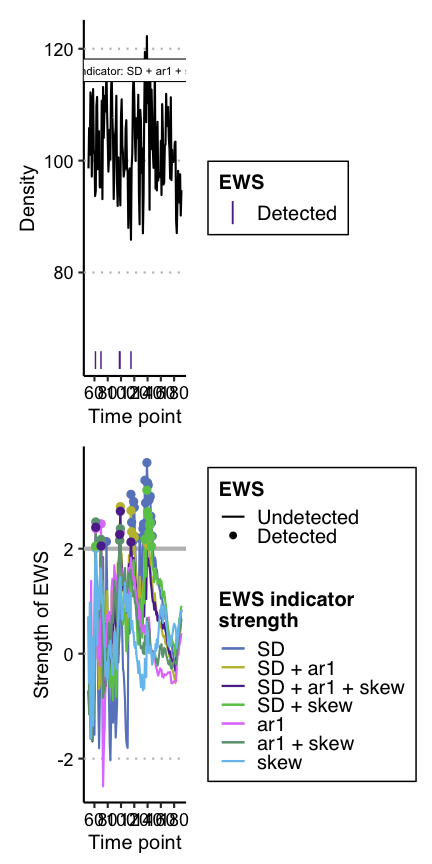Similar to the rolling window approach, EWS indicators calculated by expanding windows have exceeded the 2σ threshold for more than two consecutive time points and thus identified warnings from `time ~= 170`. However we are more confident in this conclusion as the composite metrics also display this warning (`ar1 + SD + skew`). These composite metrics simple sum the standardised individual indicator strengths together and are known to provide a more reliable signal than lone indicators (Clements & Ozgul, 2016).

### Trait information

The final contribution by `uniEWS()` is the ability to integrate multiple information sources in the assessment. For example, including body size estimates improves assessment reliability by reducing false positive rate whilst increasing the number of true positives (Clements and Ozgul 2016, Baruah et al. 2020). `uniEWS()` consequently accepts a `trait =` argument where an additional trait time series can be combined with the other abundance-based EWSs as a composite metric. This capability is only available if `method = "expanding"` and `metrics =` contains `"trait"`

``````trait_ews_eg <- uniEWS(data = pre_CODrecovery[,c(2,3)],
metrics = c("ar1","SD","trait"), #note "trait" is provided here
method = "expanding",
trait = pre_CODrecovery\$mean.size, #and here
burn_in = 15, #small burn_in due to shorter time series
threshold = 2)``````
``plot(trait_ews_eg, y_lab = "Density", trait_lab = "Mean size (g)")``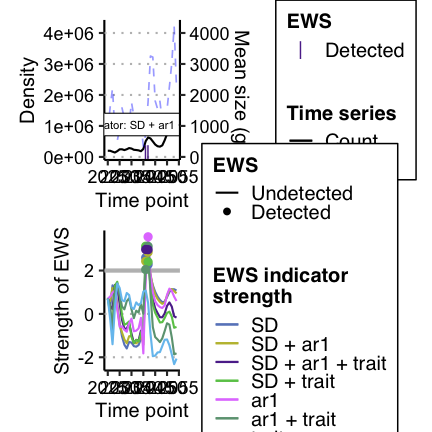## 3. Multivariate EWSs

A more powerful and informative form of EWS are multivariate EWSs. These indicators combine multiple time series/measurements of the focal system to provide a community level assessment of transition risk.

There are two primary forms of multivariate EWS, those which are averaged univariate EWS across all time series and those which are assessments made on a dimension reduction of all representative time series. A brief outline of each can be found in the following table as well as their reference code in `multEWS()` for the `metrics =` argument. See Weinans et al. (2021) for a rigorous testing of these multivariate EWSs in a simulated system.

Multivariate EWS indicator Description `multiEWS()` metric code Average or dimension reduction technique
Mean standard deviation Average variance across all time series representing the system `"meanSD"` Average
Max standard deviation The variance of the time series with the highest variance of all assessed time series `"maxSD"` Average
Mean autocorrelation at lag1 Average autocorrelation across all time series representing the system `"meanAR"` Average
Max autocorrelation at lag1 The autocorrelation of the time series with the highest autocorrelation of all assessed time series `"maxAR"` Average
Dominant MAF (maximum autocorrelation factor) eigenvalue The minimum eigenvalue of the system following MAF dimension reduction `"eigenMAF"` Dimension reduction
MAF autocorrelation The autocorrelation of the data projected on to the first MAF – i.e. the autocorrelation of the first MAF. `"mafAR"` Dimension reduction
MAF standard deviation The variance of the data projected on to the first MAF – i.e. the variance of the first MAF `"mafSD"` Dimension reduction
First PC (principal component) autocorrelation The autocorrelation of the data projected on to the first PC – i.e. the autocorrelation of the first PC `"pcaAR"` Dimension reduction
First PC standard deviation/ Explained variance The variance of the data projected on to the first PC – i.e. the variance of the first PC `"pcaSD"` Dimension reduction
First PC standard deviation/ Explained variance The variance of the data projected on to the first PC – i.e. the variance of the first PC `"pcaSD"` Dimension reduction
Dominant eigenvalue of the covariance matrix The maximum eigenvalue of the covariance matrix between all representative time series `"eigenCOV"` Neither
Maximum covariance The maximum value of the covariance matrix between all representative time series. `"maxCOV"` Neither
Mutual information The ‘amount of information’ one time series contains on another. `"mutINFO"` Neither

Using `multiEWS()` we can estimate each of these multivariate indicators in the same way as `uniEWS()` - specifying the `method =`, `winsize =` and/or `burn_in =` - but must provide a n x m dataframe/matrix of all representative time series. The first column must again be an equally spaced time sequence.

A rolling window assessment would therefore be coded as such:

``````multi_ews_eg <- multiEWS(data = pre_simTransComms[,2:7],
metrics = c("meanAR","maxAR","meanSD","maxSD","eigenMAF","mafAR","mafSD","pcaAR","pcaSD","eigenCOV","maxCOV","mutINFO"),
method = "rolling",
winsize = 50)``````
``plot(multi_ews_eg)``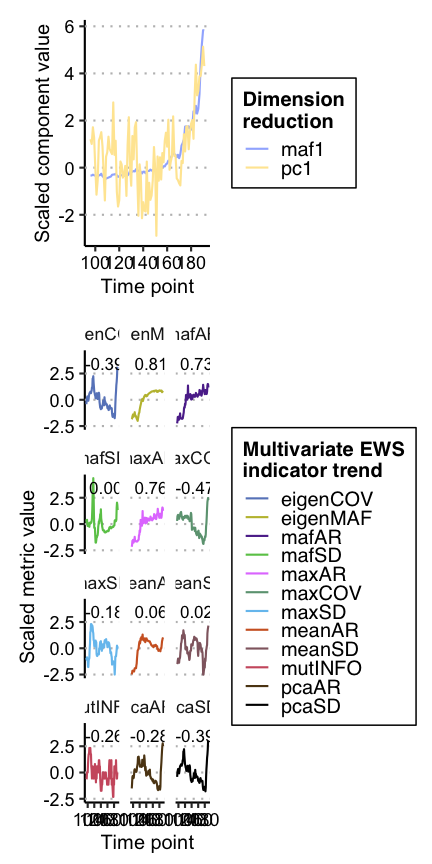Many of these indicators are postively correlated with time and therefore are ‘warnings’.

We could also use expanding windows to achieve a similar result. Note - no composite metric is computed in `multiEWS()` as it is currently unknown how combining multivariate EWS indicators influences prediction reliability.

``````multi_ews_eg2 <- multiEWS(data = pre_simTransComms[,2:7],
method = "expanding",
burn_in = 50,
threshold = 2)``````
``plot(multi_ews_eg2)``